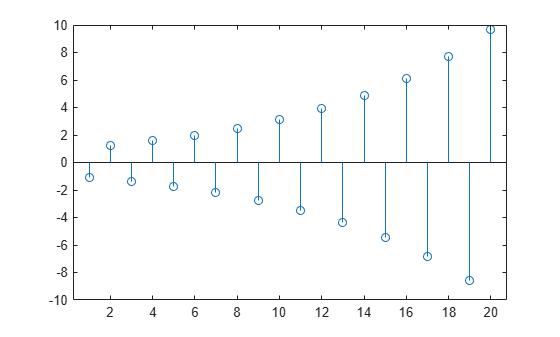# filtic

Initial conditions for transposed direct-form II filter implementation

## Syntax

``z = filtic(b,a,y,x)``
``z = filtic(b,a,y)``

## Description

````z = filtic(b,a,y,x)` finds the initial conditions, `z`, for the delays in the transposed direct-form II filter implementation given past outputs `y` and inputs `x`. The vectors `b` and represent the numerator and denominator coefficients, respectively, of the filter's transfer function.```

example

````z = filtic(b,a,y)` assumes that the input `x` is 0 in the past..```

## Examples

collapse all

Determine the zero input response of the following system: $\mathit{y}\left(\mathit{n}\right)+1.12\mathit{y}\left(\mathit{n}-1\right)=0.1\mathit{x}\left(\mathit{n}\right)+0.2\mathit{x}\left(\mathit{n}-1\right)$ with initial condition $\mathit{y}\left(-1\right)=1$. Set the numerator and denominator coefficients and the initial conditions for the output.

```b = [0.1 0.2]; a = [1 1.12]; Y = 1;```

Calculate the zero input initial conditions for the system.

`xic = filtic(b,a,Y);`

Compute the zero input response.

```yzi = filter(b,a,zeros(1,20),xic); stem(yzi)```## Input Arguments

collapse all

Transfer function coefficients, specified as vectors.

Example: `b = [1 3 3 1]/6` and `a = [3 0 1 0]/3` specify a third-order Butterworth filter with a normalized 3-dB frequency of 0.5π rad/sample.

Past output, specified as a vector. The vector `y` contains the most recent output first, and oldest output last as in

`$y=\left[y\left(-1\right),y\left(-2\right),y\left(-3\right),\dots ,y\left(-m\right)\right]$`

where `m` is `length(a)-1` (the denominator order); if `length(y)` is less than `m`, `filtic` pads it with zeros to length `m`.

Past input, specified as a vector. The vector `x` contains the most recent input first, and oldest input last as in

`$x=\left[x\left(-1\right),x\left(-2\right),x\left(-3\right),\dots ,x\left(-n\right)\right]$`

where `n` is `length(b)-1` (the numerator order). If` length(x)` is less than `n`, `filtic` pads it with zeros to length `n`

## Output Arguments

collapse all

Initial conditions, returned as a vector. The output `z` is a column vector of length equal to the larger of n and m. `z` describes the state of the delays given past inputs `x` and past outputs `y`.

## Tips

If any of the input arguments `y`, `x`, `b`, or `a` is not a vector (that is, if any argument is a scalar or array), `filtic` gives the following error message:

```Requires vector inputs. ```

## Algorithms

`filtic` performs a reverse difference equation to obtain the delay states `z`. Elements of `x` beyond `x(n-1)` and elements of `y` beyond `y(m-1)` are unnecessary so `filtic` ignores them.

The transposed direct-form II structure is shown in the following illustration.n – 1 is the filter order.

 Oppenheim, A.V., and R.W. Schafer, Discrete-Time Signal Processing, Prentice-Hall, 1989, pp. 296, 301-302.